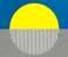Part XI

Nov.  2020

# The “Dark” Matter: Its Rarefaction Origin and Nature; Visible toDark Matter Interactions and Transformations

Three Cosmic Equations of State: Visible Matter, Dark Matter and E/M Radiation

The Possibility of Detecting

‘Visible to Dark’ Matter Transformations## Contents

1.  Introduction

2.  Properties of Three Main Equations of State

General

Equations of State

The Curvature Criterion

3.  The Elliptical Dark Matter State ( p2 /b2 + v2 / a2 = a2b2): The Dark Matter as a Rarefaction ‘Form’

General

Proposed Elliptical Equation of State for Dark Matter

Properties of the Proposed Elliptical Dark Matter

4.  The Linear State:  ‘Dark ‘ E/M Rarefied Radiation,  Does It Exist?.

5.  The Possibility of Visible-to-Dark Matter Transformations

6.Some  Cosmological Consequences

7.  Summary

## Appendix A: Formation of Visible  Hadronic  and Leptonic Matter in a Hyperbolic CompressibleShockwaveAppendix B: Outline of Compressible Flow and ShockwavesAppendix C: Transverse Waves in a Tenuous Fluid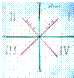### 1. Introduction

In Part X: The  Big Bang:  Visible Matter and Dark Matter Origins] [ Feb. 2020]   ,we re-examined our earlier proposal that  visible cosmic matter   (hadrons and leptons) are compression, dynamic forms which originated at the Big Bang in a hyperbolic compression shockwave, and  are also routinely  formed by the same  compression shock process in linear accelerators [2,3,4],

We then  proposed in the same document that the dark matter of the cosmos consists of  rarefaction, dynamic ‘forms’ which also originated in an elliptical rarefaction shockwave associated with the sudden inflationary expansion event which occurred immediately after the Big Bang. This would finally provide a reasonable explanation for the dark matter’s  origin, general nature, and provide a physical  reason for its apparent non-interaction with our visible matter world, ours being compressive and the dark world being rarefied.

We now continue our examination of rarefaction compressibility to yield more detail on the nature of the Dark Matter World including the possibility that a form of ‘dark radiation” exists and is its ‘illumination’, as well as a proposal for a visible to dark matter transformations.

First, we put this in context with a look at three cosmic Equations of State.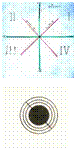#### 2.0    Properties of Three Main Equations of State

##### 2.1 General

Equations of State are equations which link the thermodynamic ‘state’ variables of pressure, p, specific volume (v = 1/ρ) and temperature  T. [www.enegycompressibility.info : Part VIII : Equations of State for Cosmic Fields: [Ref 13].

These “states”  are the dynamic, compressible entities  in which waves travel and in which shock waves of either compression or of rarefaction form and produce elementary forms or particles of matter, visible or ‘dark as the case may be, but which so far have appeared to be almost completely separate and noninteracting .

For example,  we have (a) the Ideal Gas Law as the hyperbolic  equation of state describing the production of  visible matter [ pv = const. = RT] : (b)  the elliptical equation of state  [p2/b2 + v2/a2 = a2b2 = const] which we propose to describe the dark matter of the cosmos., and (c)  the linear equation [p = ±Av]  which  will be seen to describe both Maxwell’s Electromagnetic waves, as well as newly proposed ‘dark’ electromagnetic waves to irradiate  the dark matter world.

These three equations of state are depicted on pressure  p, versus –specific volume  ( v = 1/ϼ)  diagrams, as in Fig. 1, Quadrant 1.

I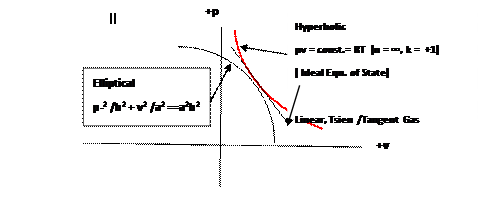Figure 1:  Equations of State for Visible Matter, Dark Matter and Linear Waves

2.2 Equations of State and Wave Types Supported by Each State:

(1)The hyperbolic state supports compression waves only; rarefaction waves die out but compression waves of finite amplitude grow to compression  shocks. This state describes our visible or Hubble world in which the elementary particles of matter form in compression shocks.

The properties of our ordinary visible matter are, of course, well known and in voluminous detail. The state is one of condensed energy forms, i.e:  elementary mass-particles, atoms, molecules.

For visible matter, the equation of state is  hyperbolic: [ pv = RT = constant].

The visible matter interacts intimately with a linear wave state via the electro- magnetic force and via absorption and emission of condensed electromagnetic radiation  ( i. e of photons).

(2)   The linear state supports stable waves of both compression and rarefaction. Shocks cannot occur.  The compression linear waves correspond to the stable E/M waves of our visible cosmos.

The  linear equation of state  [p  = ±Av  ±B]  supports the condensed photons of electromagnetic radiation and its interaction with visible matter.  Theoretically this equation also supports rarefaction waves  and so the possibility of  rarefied  E/M radiation arises.

(3)   The elliptical state, we now  propose,  pertains to the dark matter. This state supports rarefaction waves only. Waves of finite amplitude grow to form rarefaction shocks  in which we propose  dark matter ‘forms’ emerge to produce a rarefied  kind of matter.

(Since the assigned elliptical equation of state for  the dark matter supports only rarefaction waves and rarefaction shocks, any ‘forms’ would then necessarily be of  ‘rarefied  matter’, whatever this may mean. One might suspect that, because in our scheme the dark matter's rarefied state is opposite to the compressive state of  our visible matter, that this might  now explain the lack of  any observed interaction of dark matter with condensed visiblematter, as is currently the case.

Note: We should point out here that the three curves in Fig. 1  are for illustration of  equation type only. That is to say, the relative sizes of the areas ( pv) under each curve ( pv represents an energy amount) are  not representative of actually existing relative total energies; this would require expert assessment.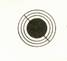2.3 The Curvature Criterion for  the Three Equations of State    [Ref. (5)  Shapiro. Vol. 1]

Pulses or changes in flow rate in compressible fluids cause pressure fluctuations which result

in traveling disturbances called   pressure waves.  These waves can be of either compression or rarefaction depending on the physical nature of the compressible fluid as expressed in its equation of state. Waves in material gases of visible matter (hyperbolic equation of state) are always compression while rarefaction waves are damped and die out. For the elliptical state the reverse is the case and rarefaction waves prevail.

If the wave amplitude is ‘infinitely’ small, the waves are stable and are called ‘acoustic waves’. If the wave amplitude is finite to begin with then, in both the hyperbolic and elliptical states, the waves grow rapidly to form shocks or shock wave, respectively of compression and of rarefaction.

The criterion for wave behavior is the curvature of the equation of state [d2p/dv2 ]   on the pv diagram.

PFig. 2.  Hyperbolic and Elliptical  Equations of State with opposite   curvature

1.                  If the curvature is positive [d2p/dv2 > 0] as in the hyperbolic, visible matter case, then the compression waves are non-linear and steepen with time to form compression shocks; rarefaction waves die out.

2.                  If the curvature is negative [d2p/dv2 ‹ 0], as in the elliptical dark matter, then their rarefaction waves are non-linear and steepen with time to form rarefaction shocks while compression waves die out.

[Note: In all states, if the pressure disturbances are of infinitesimally low amplitude then stable waves of either compression or rarefaction called acoustic waves can persist without growing to shocks].

3.                  In the case where the curvature is zero [d2p/dv2 = 0], the pv curve is linear , i.e. it is a straight line, [p = ±Av ±B]  and waves of both compression or rarefaction, and of any amplitude large or small, are all stable and can propagate unchanged. This linear case can also be visualized as the tangent case linking the hyperbolic and elliptical state equations.

To repeat, waves large or small, in this linear state, are all stable and all can propagate unchanged. Growth to shock waves is not possible in the linear case. [Ref.  (5)  Shapiro. Vol. 1]

###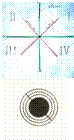3. The Elliptical Dark Matter State ( p2 /b2 + v2 / a2 = a2b2) : The Dark Matter Seen asRarefaction 'Forms' or Rarefaction ‘Substances’

#### 3.1 General

As we have shown in Part X, the formation of the elementary particles of our visible universe

can  be described by compression shocks in  the hyperbolic  state [pv = constant] occurring  in the Big Bang event.[1,4].We now  propose that the dark  matter’s elliptical  state should  produce rarefaction shocks and rarefaction forms, and that this rarefaction property --as opposed to compression properties--  may then  explain both the dark matter’s  invisibility  and its apparent non-interaction with visible forms of matter, except by way of gravity.

Our elliptical equation of state for the dark matter is :

## P2 /b2   - v2/a2  = a2b2=

/.

If the curvature is positive   [ d2p/dv2 › 0] as in material gases, then hyperbolic state compression  waves steepen to form compression shocks and rarefaction waves die out.

If the curvature is negative [d2p/dv2 ‹ 0], as in the proposed elliptical dark matter case, then rarefaction waves would steepen with time to form rarefaction shocks while compression waves would die out.

P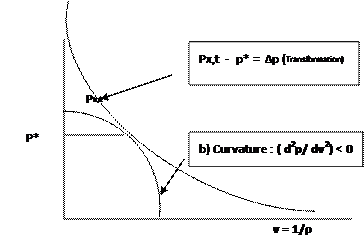Fig. 3.  Hyperbolic and Elliptical  Equations of State with opposite   curvatureWe understand a great deal about ordinary hyperbolic matter ( 4.9%  of the cosmos),  but very little about the much more abundant dark matter (27%).  The dark matter apparently occurs everywhere and has a strong influence on the structure, dynamics and the physical evolution of the cosmos. It does not appear to interact with ordinary matter, nor with electromagnetic radiation. Its interaction with our ordinary matter is apparently only by gravitation, although there is some thought that the dark matter may interact very weakly with matter by some application of the weak nuclear force.

Some other explanatory candidates have been that the dark matter is some new kind of unknown baryonic elementary particle, or involves weakly interacting WIMPS. Currently theories also distinguish between Cold, Warm or Hot Dark Matter.

However, ln this Report we have assigned to the dark matter a rarefaction nature, with its cosmic origin being at a strong rarefaction shock wave occurring in the sudden and massive inflationary expansion that occurred right after the Big Bang’s enormous compression state.

As a background to this new rarefaction shockwave proposition, we mention that we have earlier proposed that the ordinary matter’s elementary particles are routinely formed in compression shockwaves in linear accelerators, and that this is verified in the predicted mass ratios which are in good agreement with  the experimentally measured  mass ratios.    [Appendix A: Formation of Visible Baryonic Matter in a Hyperbolic Compressible Shockwave]

IFigure 1:  Equations of State for Visible Matter, Dark Matter and Linear Waves4.0 The Linear State: Dark E/M Rarefied Radiation,  Does It Exist?

The linear state equation [ p = ± Av ±B] is pre-eminently a stable wave equation.   These linear waves can be of either compression or of rarefaction, but all are stable, propagating without change in amplitude, so that shocks are impossible. The linear compression waves interact with our compression visible matter as observable E/M photonic radiation..

It seems reasonable therefore to propose then that there may also exist linear ‘dark’ rarefaction wave forms i.e.  ‘rarefied photons’ which parallel  the linear E/M compression photons which interact with our compression visible  matter as E/M radiation.

A further question which arises is whether rarefied dark matter can interact with rarefied linear radiation? This would parallel the interaction of visible compression matter with the Maxwell’s compressed photonic electromagnetic linear radiation [ 17].  If so, the resulting ‘dark matter’ and its associated ‘dark’ radiation’ World would lie all about us. It is known through its gravitation to be five times as abundant as our own visible world, but it is as yet undetectable by any known means apart from its mentioned gravitational attraction. [

This then raises the possibility that a ‘dark linear E/M  to dark matter  interaction’ may  be detectible by some means yet to be discovered.

To summarize: The linear equation of state  [p = ±AV] is here  taken as describing sets of linear waves, not only of photonic compression but of ‘photonic’ rarefaction as well.  And so, we may now explore the possibility that “dark” leptons expressing ‘dark’ electromagnetism may also exist.

The proposal is a valid scientific proposition. It does however as yet lack some testable effects. We suggest that such effects may lie in some small numerical discrepancies in the experimental data of existing cosmological and quantum experimental testing.5.0 The Possibility of Visible to Dark Matter Transformations:   Is the Concept of Dark Energy Necessary?

Astronomical observations, of a large increase in the Cosmic Microwave Background Radiation ( CMB) discovered \around 1998. was interpreted as showing the universe to be undergoing an accelerated expansion. In explanation,  the concept of an all pervasive “dark energy” exerting an expansion pressure was introduced. The amount of this new hypothetical energy needed to explain the CMB increase was enormous, constituting some 69% of the known universe compared with only 4.9% for the visible universe.

Towards a simplification,  we now propose  a transformation  from World A matter to World B matter.

## A Possible Transformation from Visible Matter to the “ Dark Matter”

. This transformation  of World A visible matter to World B dark matter A  would logically take place at a common tangential point having a  common critical pressure p* ( Fig. 3.).